Day 5 MPM 2 D Review of Essential

• Slides: 15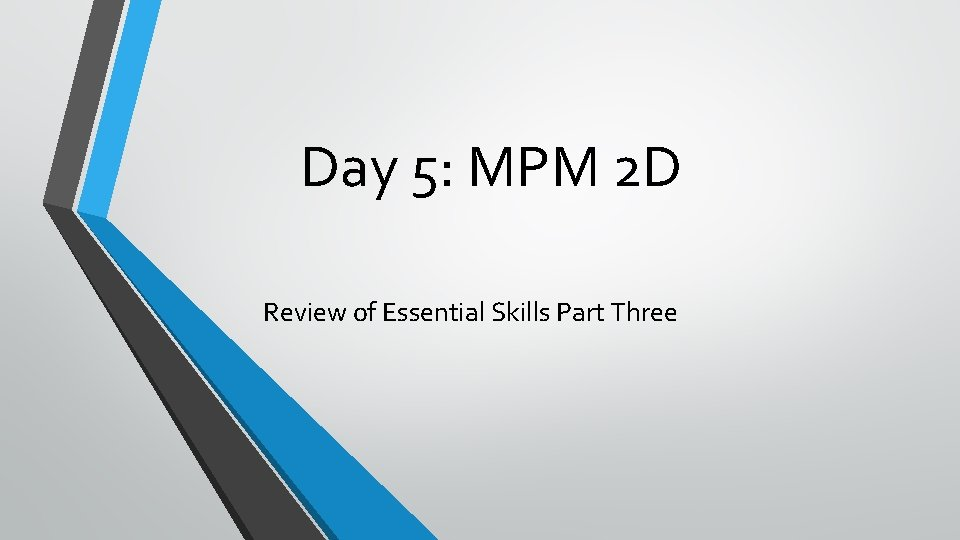Day 5: MPM 2 D Review of Essential Skills Part Three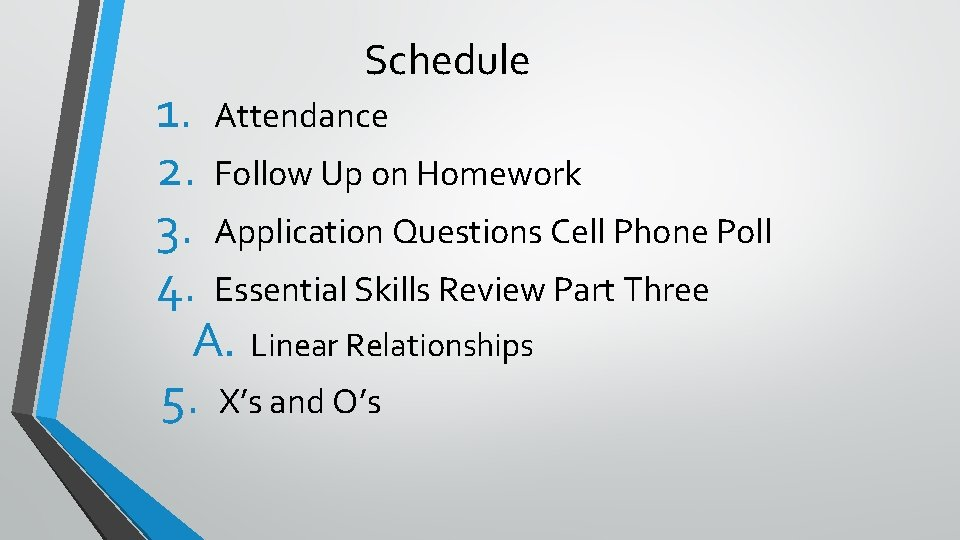Schedule 1. 2. 3. 4. Attendance 5. X’s and O’s Follow Up on Homework Application Questions Cell Phone Poll Essential Skills Review Part Three A. Linear RelationshipsQuestions from Homework?Cell Phone PollHow is the material so far? Close your eyes and respond using the scale below: This stuff is easy/I am very comfortable Some stuff is clear/I am still unsure about some things I am lost/Have no idea what is going on/I need some help reviewing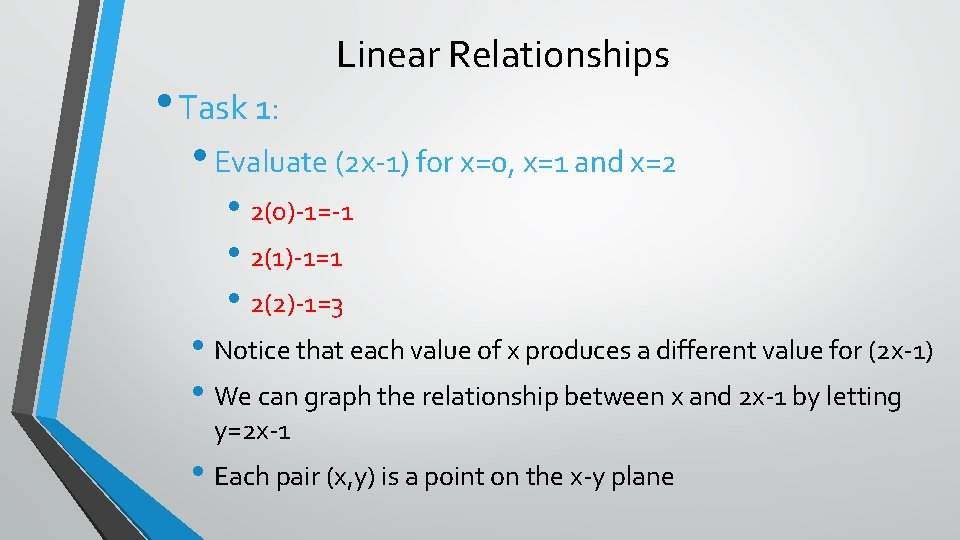• Task 1: Linear Relationships • Evaluate (2 x-1) for x=0, x=1 and x=2 • 2(0)-1=-1 • 2(1)-1=1 • 2(2)-1=3 • Notice that each value of x produces a different value for (2 x-1) • We can graph the relationship between x and 2 x-1 by letting y=2 x-1 • Each pair (x, y) is a point on the x-y plane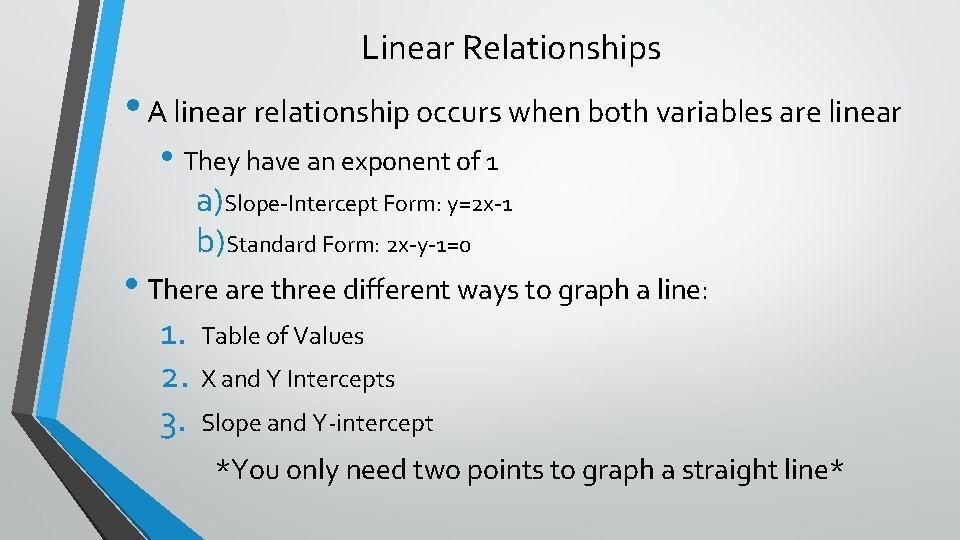Linear Relationships • A linear relationship occurs when both variables are linear • They have an exponent of 1 a)Slope-Intercept Form: y=2 x-1 b)Standard Form: 2 x-y-1=0 • There are three different ways to graph a line: 1. Table of Values 2. X and Y Intercepts 3. Slope and Y-intercept *You only need two points to graph a straight line*Graphing Using a Table of Values y=(2 x-1) -5 -3 -1 1 3 Y-axis x -2 -1 0 1 2 4 3 2 1 0 -3 -2 -1 -1 -2 -3 -4 -5 -6 0 1 2 X-Axis 3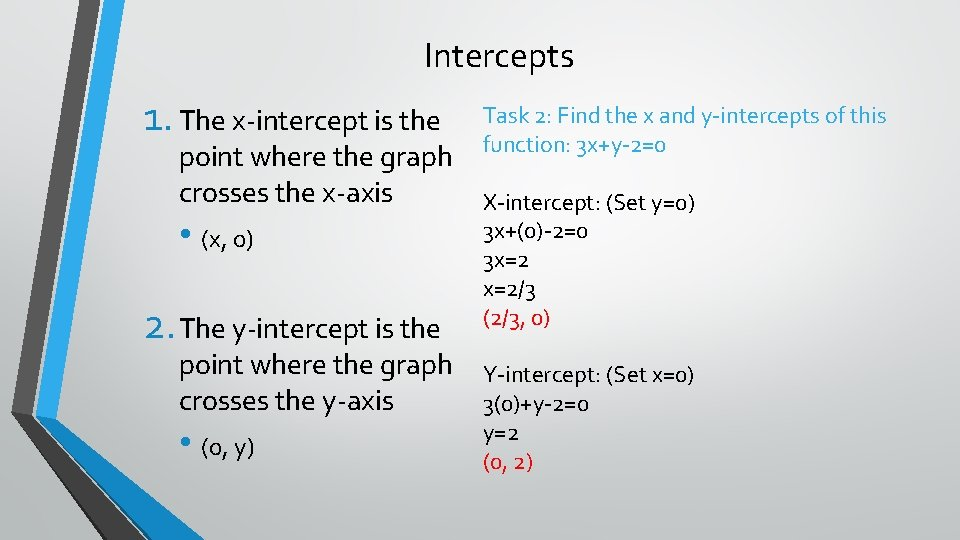Intercepts 1. The x-intercept is the point where the graph crosses the x-axis • (x, 0) 2. The y-intercept is the point where the graph crosses the y-axis • (0, y) Task 2: Find the x and y-intercepts of this function: 3 x+y-2=0 X-intercept: (Set y=0) 3 x+(0)-2=0 3 x=2 x=2/3 (2/3, 0) Y-intercept: (Set x=0) 3(0)+y-2=0 y=2 (0, 2)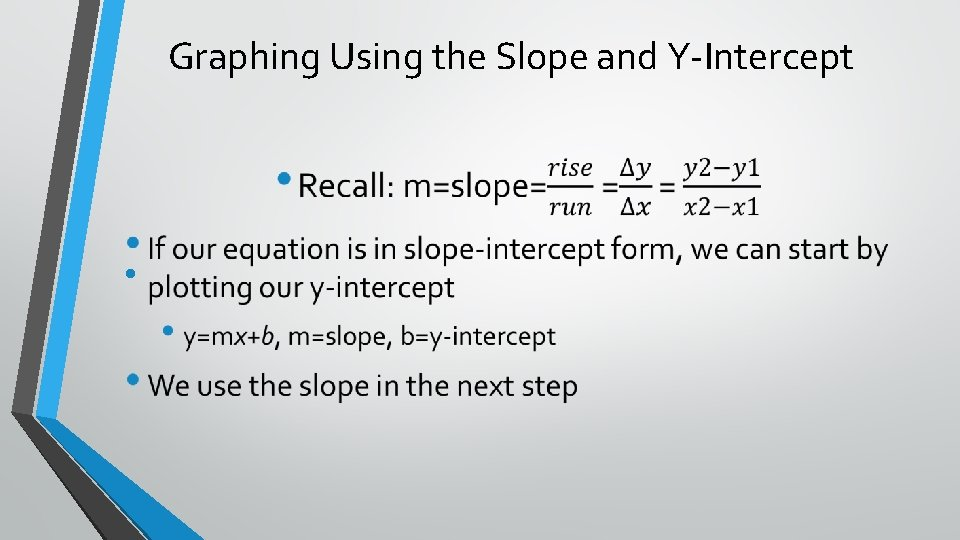Graphing Using the Slope and Y-Intercept •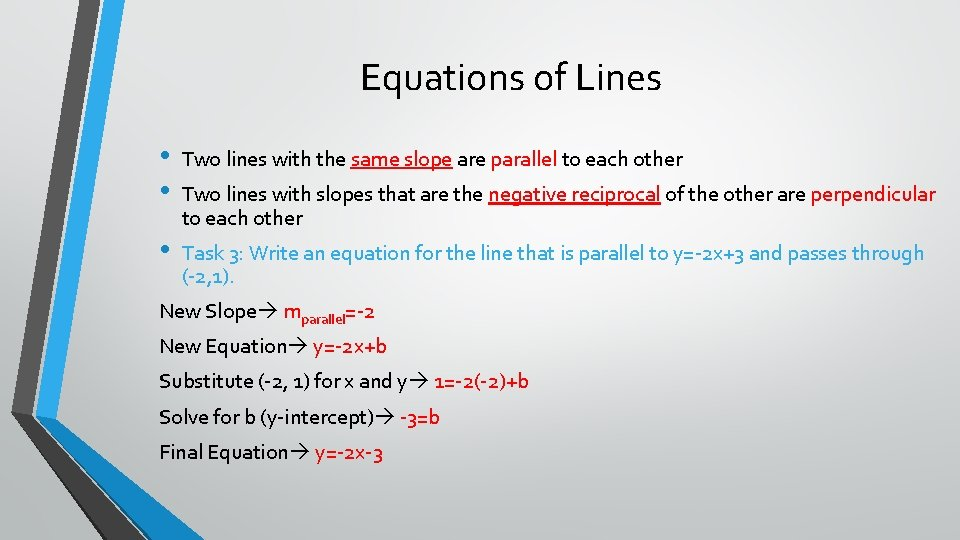Equations of Lines • • Two lines with the same slope are parallel to each other • Task 3: Write an equation for the line that is parallel to y=-2 x+3 and passes through (-2, 1). Two lines with slopes that are the negative reciprocal of the other are perpendicular to each other New Slope mparallel=-2 New Equation y=-2 x+b Substitute (-2, 1) for x and y 1=-2(-2)+b Solve for b (y-intercept) -3=b Final Equation y=-2 x-3Class Work • P. 26 #1 -5 (Odd only) • P. 28 #1, 2(bd), 3, 6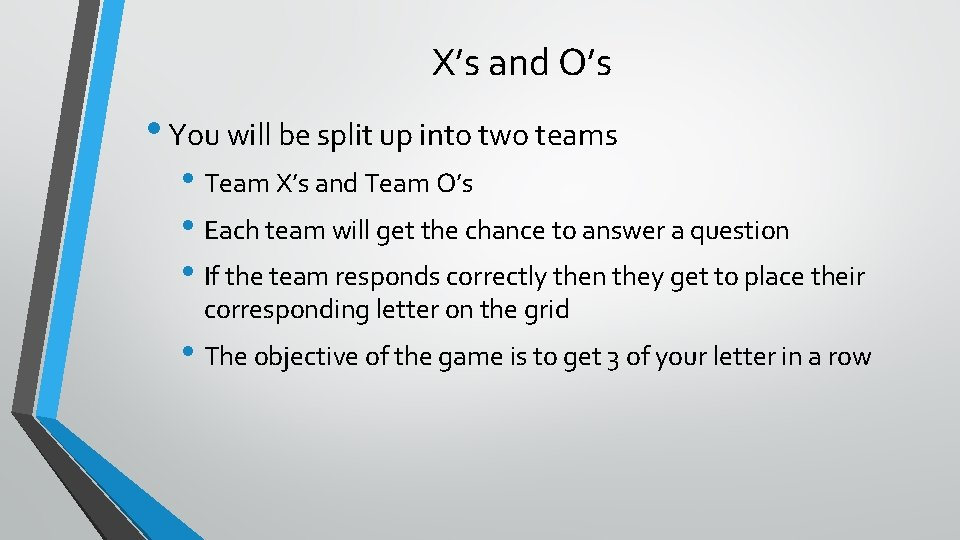X’s and O’s • You will be split up into two teams • Team X’s and Team O’s • Each team will get the chance to answer a question • If the team responds correctly then they get to place their corresponding letter on the grid • The objective of the game is to get 3 of your letter in a row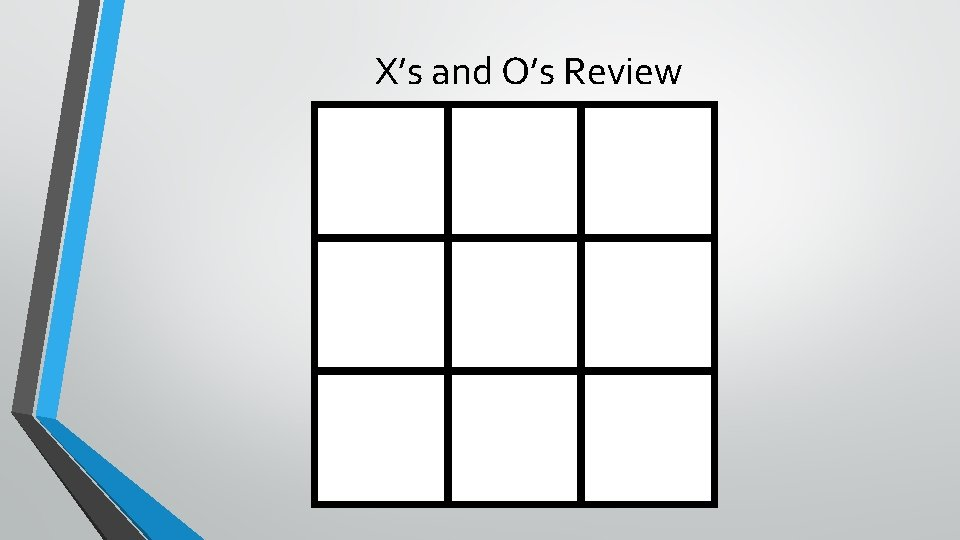X’s and O’s Review